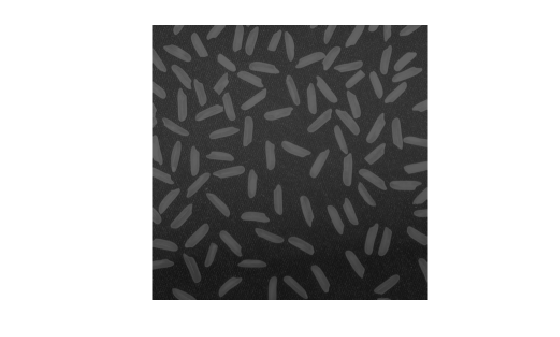# imdivide

Divide one image into another or divide image by constant

## Syntax

``Z = imdivide(X,Y)``

## Description

example

````Z = imdivide(X,Y)` divides each element in the array `X` by the corresponding element in array `Y` and returns the result in the corresponding element of the output array `Z`.```

## Examples

collapse all

This example shows how to divide two `uint8` arrays.

```X = uint8([ 255 0 75; 44 225 100]); Y = uint8([ 50 50 50; 50 50 50 ]);```

Divide each element in `X` by the corresponding element in `Y`. Note that fractional values greater than or equal to 0.5 are rounded up to the nearest integer.

`Z = imdivide(X,Y)`
```Z = 2x3 uint8 matrix 5 0 2 1 5 2 ```

Divide each element in `Y` by the corresponding element in `X`. Note that when dividing by zero, the output is truncated to the range of the integer type.

`W = imdivide(Y,X)`
```W = 2x3 uint8 matrix 0 255 1 1 0 1 ```

Read a grayscale image into the workspace.

`I = imread('rice.png');`

Estimate the background.

`background = imopen(I,strel('disk',15));`

Divide out the background from the image.

`J = imdivide(I,background);`

Display the original image and the processed image.

`imshow(I)````figure imshow(J,[])```Read an image into the workspace.

`I = imread('rice.png');`

Divide each value of the image by a constant factor of 2.

`J = imdivide(I,2);`

Display the original image and the processed image.

`imshow(I)````figure imshow(J)```## Input Arguments

collapse all

First array, specified as a numeric array or logical array of any dimension.

Second array (divisor) to be divided from `X`, specified as a numeric or logical array of the same size and class as `X`, or a numeric scalar of type `double`.

## Output Arguments

collapse all

Quotient, returned as a numeric array of the same size as `X`. `Z` is the same class as `X` unless `X` is logical, in which case `Z` is data type `double`. If `X` is an integer array, elements of the output that exceed the range of the integer type are truncated, and fractional values are rounded.

## Version History

Introduced before R2006a